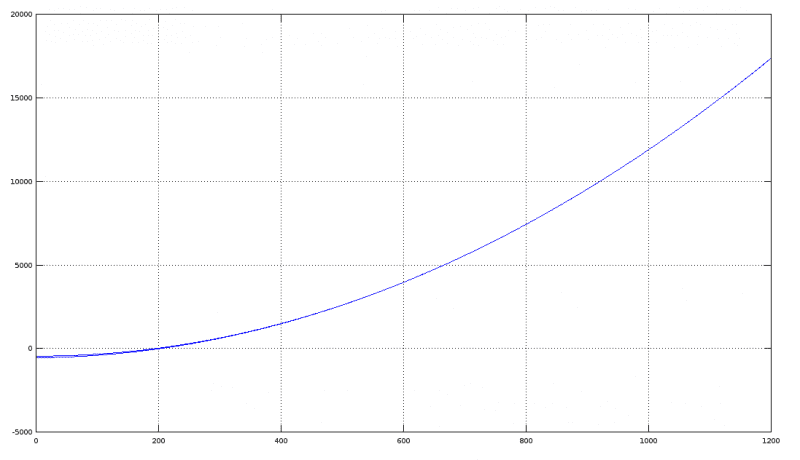# Interpolation and linear algebra

## Homework Statement

As a string in my program.

## Homework Equations

Solving a system with the forward phase of row echelon reduction and a consecutive back substitution.
All done by numpy here. (The book suggested MATLAB, etc).

## The Attempt at a Solution

Python:
import numpy

""" In a wind tunnel experiment, the force on a projectile due to air
resistance was measured at different velocities:
Velocity (100 ft/sec) 0 2.00 4.00 6.00 8.00 10.00
Force (100 lb)        0 2.90 14.8 39.6 74.3 119.0
Find an interpolating polynomial for these data and estimate the force on the
projectile when the projectile is traveling at 750 ft/sec.
Use p(t) = a0 + a1*t + a2*t^2 + a3*t^3 + a4*t^4 + a5*t^5.
What happens if you try to use a polynomial of degree less than 5?
(Try a cubic polynomial, for instance.)
"""

make_row_for_t = lambda t: [t ** n for n in range(6)]

A = numpy.array([make_row_for_t(t * 100) for t in range(0, 11, 2)])
B = numpy.array([[n] for n in [0, 290, 1480, 3960, 7430, 11900]])

coefficients = numpy.linalg.solve(A, B)

p = numpy.polynomial.Polynomial(coefficients.flatten())

print_p = lambda x: print("{:<8} = {}".format("p(" + str(x) + ")", p(x)))

for n in range(0, 1100, 200):
print_p(n)

print_p(750)

Code:
p(0)     = 0.0
p(200)   = 290.0000000000015
p(400)   = 1480.0000000000023
p(600)   = 3960.0000000000036
p(800)   = 7430.000000000004
p(1000)  = 11900.00000000001
p(750)   = 6483.837890625005

Did it work? Yes. (Just get the python3 and python3-numpy packages under Linux and it should run in your machine too.)

The book does not have the answer, but mine seems OK, I wanted to get some review on what I did as I am new to numpy and interpolation and thought I would get some useful advice or even corrections.

## Answers and Replies

The interpolation requires a polynomial in x5, as you have six data points. For 750 fps, you get 6483,83 lbs

With a smaller polynomial, and if you want to consider all the points, you would have to conform yourself with a best-fit (that, in this case, is quite good with a third-degree polynomial...).Since you are asked for the resistance at 750 fps, the sensible thing to do, in my opinion, is to take the last four points and to obtain the interpolation, third-degree polynomial.

SteamKing
Staff Emeritus
Science Advisor
Homework Helper
Very few things are interpolated well using polynomials higher than third order. You fight round-off errors and then the polynomial will want to oscillate. Most data interpolate quite well with either parabolas or cubics.

Interesting. Thank you for the answers, will have a somewhat decent graph of the cubic soon. Maybe will compare it with the fifth degree polynomial. My first thought was that really small coefficients and bigger polynomials would just make the interpolation more and more accurate.

SteamKing
Staff Emeritus
Science Advisor
Homework Helper
Interesting. Thank you for the answers, will have a somewhat decent graph of the cubic soon. Maybe will compare it with the fifth degree polynomial. My first thought was that really small coefficients and bigger polynomials would just make the interpolation more and more accurate.

If the coefficients of the higher powers of your interpolating polynomial are really small, that can also be an indication that a lower order polynomial will interpolate the data just fine.

The interpolation requires a polynomial in x5, as you have six data points. For 750 fps, you get 6483,83 lbs

With a smaller polynomial, and if you want to consider all the points, you would have to conform yourself with a best-fit (that, in this case, is quite good with a third-degree polynomial...).Since you are asked for the resistance at 750 fps, the sensible thing to do, in my opinion, is to take the last four points and to obtain the interpolation, third-degree polynomial.
p(t) = 2.0833e-07*t^3 + 0.012*t^2 + 0.24167*t^1 - 550 // using the last 4 points.
p(t) = 0.0125*t^2 - 0.15*t^1 - 450 // using the last 3 points.
Nice that the graphics (in the region of interest) almost coincide.